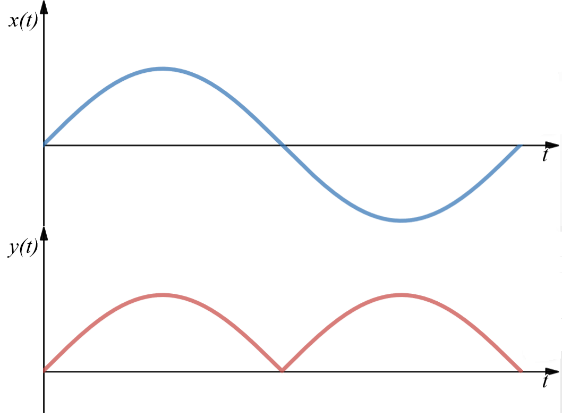# Abs

Description of the Abs component in Schematic Editor, which outputs the absolute value of an input signal.

## Description

If the input signal of Abs component is given by x(t), the output signal, y(t), is given by:

y(t)=|x(t)|

Figure 1 below illustrates the behavior of the output, y(t), of the Abs component if an arbitrary signal, x(t), is connected to its input:Figure 2. Output signal of the abs component for an arbitrary input

## Ports

• Input port (in)
• Supported types: uint, int and real.
• Vector support: yes.
• Output port (out)
• Supported types: uint, int and real.
• Output type is inherited from the input signals.
• Vector support: yes.
• Vector length is inherited from input signal.

## Properties• Execution rate:
• Type in the desired signal processing execution rate. This value must be compatible with other signal processing components of the same circuit: the value must be a multiple of the fastest execution rate in the circuit. There can be up to four different execution rates, but they must all be multiple of the basic simulation timestep. To specify the execution rate, you can use either decimal (e.g. 0.001) or exponential values (e.g. 1e-3) in seconds. Alternatively, you can type in ‘inherit’ in which case the component will be assigned execution rate based on the execution rate of the components it is receiving input from.